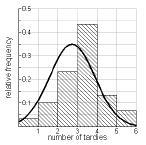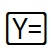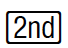Home > INT3 > Chapter 4 > Lesson 4.4.1 > Problem4-105

4-105.

Do you remember the problem highlighting students tardy to Ms. Greene’s class? Now that you have investigated how to create and use a normal distribution, model the number of tardy students Ms. Greene actually recorded using a normal distribution. The data Ms. Greene gathered for $30$ days is shown in the table below. Homework Help ✎

 Number of tardy students per day $2$ $1$ $2$ $3$ $5$ $4$ $3$ $3$ $0$ $3$ $3$ $3$ $1$ $2$ $3$ $3$ $4$ $2$ $3$ $3$ $4$ $3$ $3$ $2$ $4$ $2$ $2$ $3$ $5$ $1$ checksum $82$

You will need to use your calculator to complete this problem.

1. On your calculator, recreate the relative frequency histogram for the number of tardy students per day that is shown in problem 4‑76.2. What are the mean and standard deviation of the number of tardy students per day?

3. On your calculator, model the data with a normal distribution by pressingand entering[DISTR] normalpdf(X, mean, standard deviation). Sketch the model with the histogram.

4. According to your model, on what percentage of days were four or more people tardy? Shade this proportion on a new sketch of the model, and calculate the proportion using normalcdf(lower, upper, mean, standard deviation) on your calculator.

$\approx13.4\%$
Be sure to sketch and shade the model.

5. Assume that the last $30$ days in Ms. Greene’s class were representative of the $180$ days in the whole school year. According to your model, how many days this year can Ms. Greene expect four or more tardy students?

normalcdf(4, 10^99, 2.7333, 1.1427)